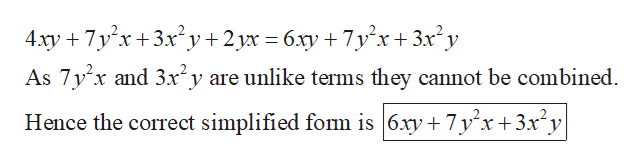Decide if the following attempts to combine like terms arecorrect. If a mistake was made, say what it was and correct it.a. 4xy + 7y2x + 3x2y + 2yx = 6xy + 10x3y3b. (2x2 + 1) + (3x + 7) + (x2 − 8x)=3x2 − 5x + 8

Question

Decide if the following attempts to combine like terms are
correct. If a mistake was made, say what it was and correct it.

a. 4xy + 7y2x + 3x2y + 2yx = 6xy + 10x3y3

b. (2x2 + 1) + (3x + 7) + (x2 − 8x)=3x2 − 5x + 8

Step 1

The error in the simplified form  ...help_outlineImage Transcriptionclose4xy 7y x 3x2y +2yx 6.y7yx+3x2y As 7y x and 3x2y are unlike terms they cannot be combined. Hence the correct simplified fom is |6.xy +7y x+3x2y fullscreen

Want to see the full answer?

See Solution

Want to see this answer and more?

Our solutions are written by experts, many with advanced degrees, and available 24/7

See Solution
Tagged in

Algebra